Question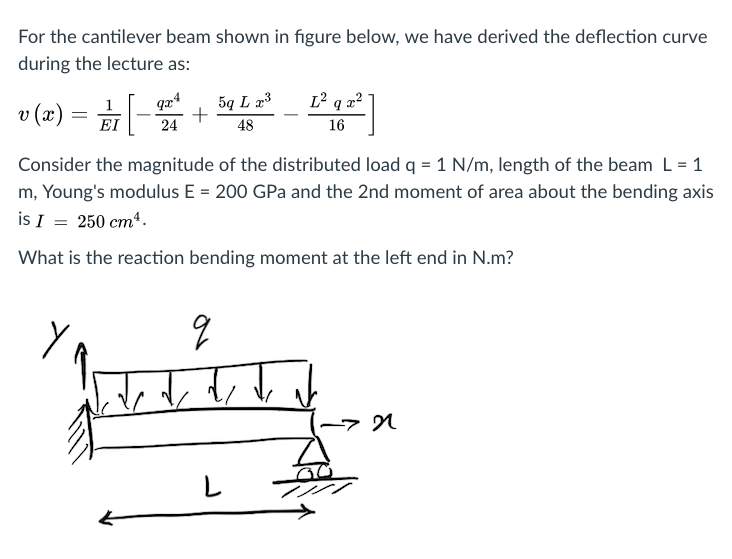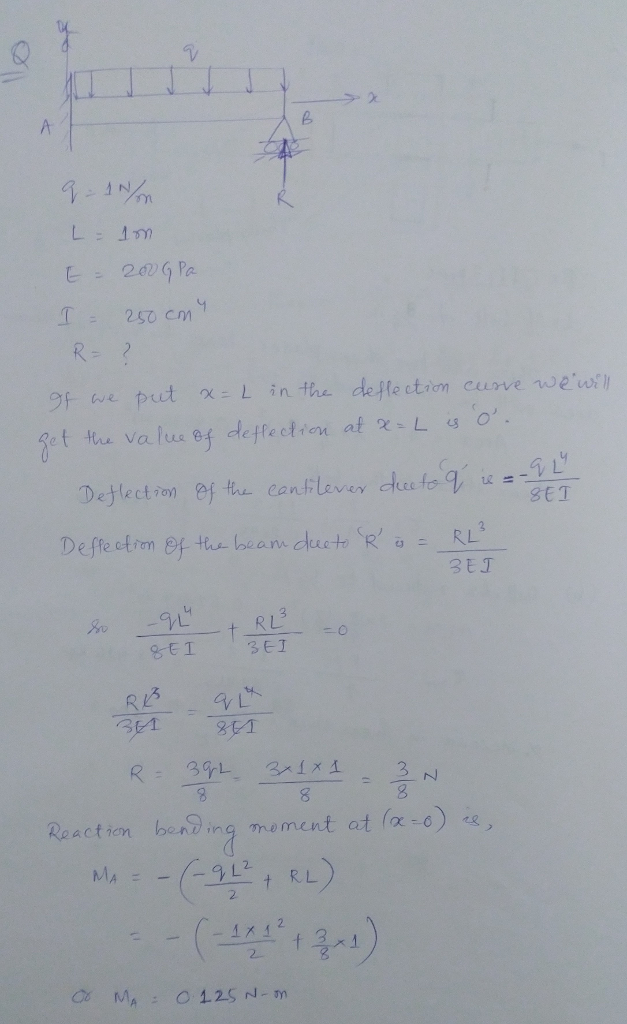#### Earn Coins

Coins can be redeemed for fabulous gifts.

Similar Homework Help Questions
• ### Q5. The cantilever beam, AC, is subjected to the load case shown in Figure 5. For the loading shown, do the following: [10 Marks] a) Calculate the magnitude and direction of the reactions at A b) Usi...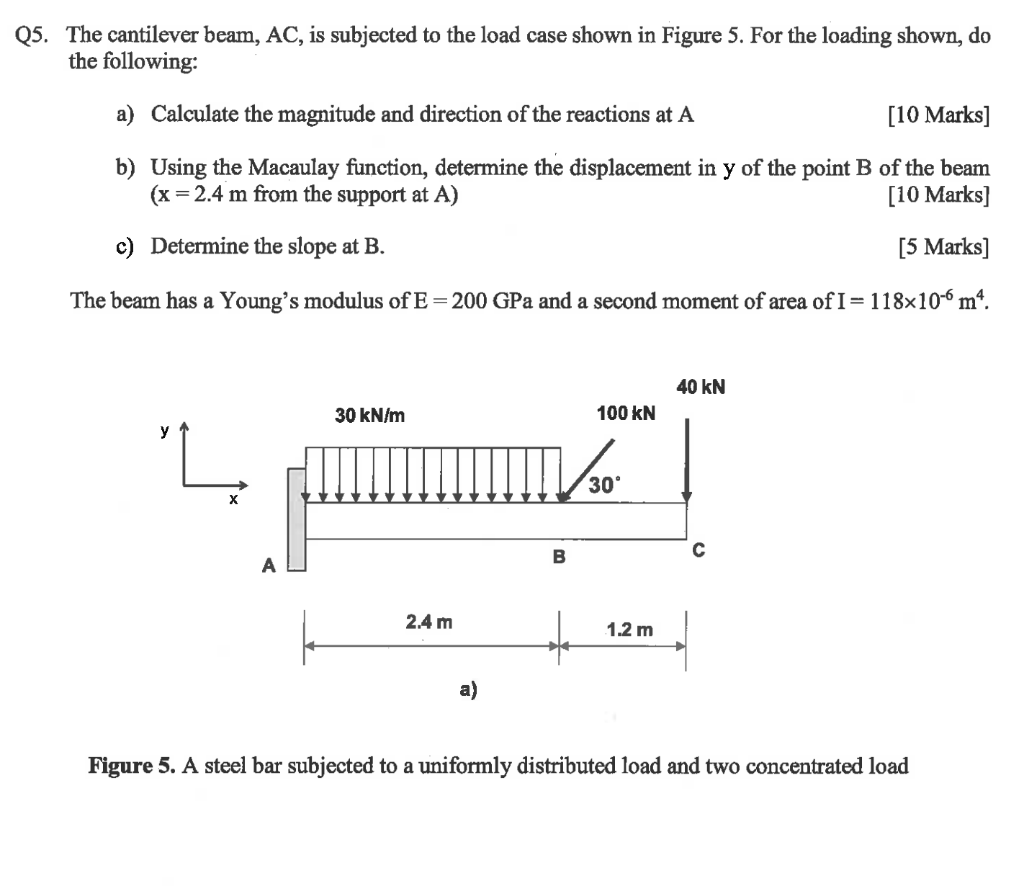Q5. The cantilever beam, AC, is subjected to the load case shown in Figure 5. For the loading shown, do the following: [10 Marks] a) Calculate the magnitude and direction of the reactions at A b) Using the Macaulay function, determine the displacement in y of the point B of the beam (x 2.4 m from the support at A) [10 Marks] c) Determine the slope at B. [5 Marks] The beam has a Young's modulus of E-200 GPa and...

• ### DE = 29 Question 4: Indeterminate Beam Design and Deflection A 2014-T6 aluminium cantilever beam is...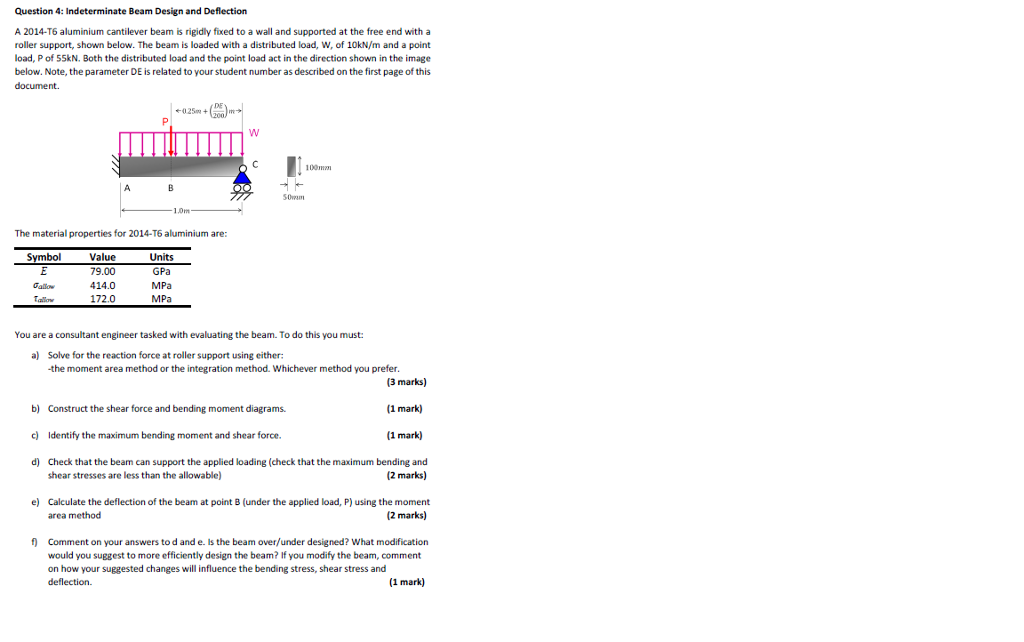DE = 29 Question 4: Indeterminate Beam Design and Deflection A 2014-T6 aluminium cantilever beam is rigidly fixed to a wall and supported at the free end with a roller support, shown below. The beam is loaded with a distributed load, W, of 10kN/m and a point load, P of 55kN. Both the distributed load and the point load act in the direction shown in the image below. Note, the parameter DE is related to your student number as described...

• ### A cantilever beam is shown in the figure below. Using the second-order integration method (moment-curvature equation):...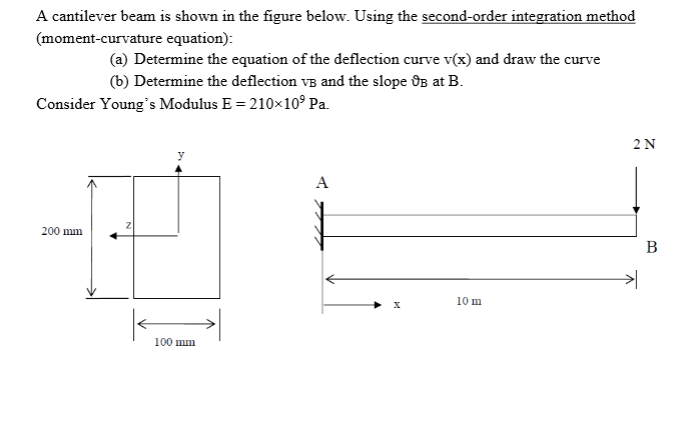A cantilever beam is shown in the figure below. Using the second-order integration method (moment-curvature equation): (a) Determine the equation of the deflection curve v(x) and draw the curve (6) Determine the deflection ve and the slope OB at B. Consider Young's Modulus E = 210x10° Pa. 2N А 200 mm B > 10 m 100 mm

• ### 8. The cantilever beam in Figure Q8 subjects to concentrated loading. The cross section geometry gives...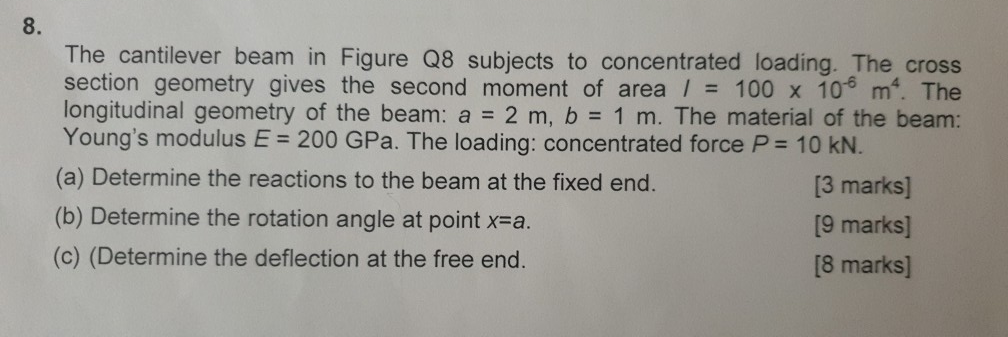8. The cantilever beam in Figure Q8 subjects to concentrated loading. The cross section geometry gives the second moment of area / 100 x 10 m. The longitudinal geometry of the beam: a 2 m, b 1 m. The material of the beam: Young's modulus E 200 GPa. The loading: concentrated force P 10 KN. (a) Determine the reactions to the beam at the fixed end. (b) Determine the rotation angle at point x-a (c) (Determine the deflection at the...

• ### A cantilever beam of a channel section is loaded at its half-length, as shown in Figure Q2. The Young's modulus of the material is 200 GPa. Determine the deflection at the free end. [12.5 marks]...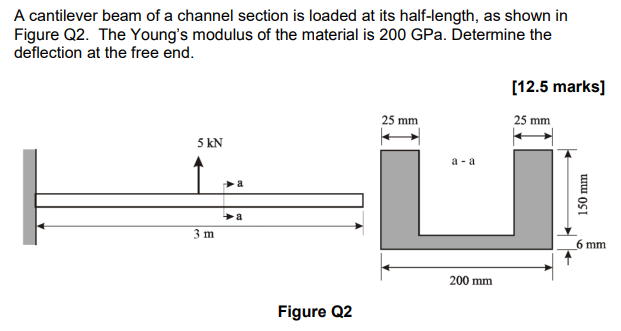A cantilever beam of a channel section is loaded at its half-length, as shown in Figure Q2. The Young's modulus of the material is 200 GPa. Determine the deflection at the free end. [12.5 marks] 25 mm 25 mm 5 kN a -a 少a 6 mm 200 mm Figure Q2 A cantilever beam of a channel section is loaded at its half-length, as shown in Figure Q2. The Young's modulus of the material is 200 GPa. Determine the deflection at...

• ### A rectangular cross section at a location along a beam in bending is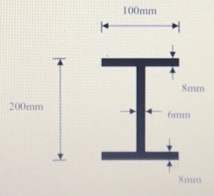(a). A rectangular cross section at a location along a beam in bending is acted upon by a bending moment and a shear force. The cross section is $$120 \mathrm{~mm}$$ wide, $$300 \mathrm{~mm}$$ deep and is orientated such that it is in bending about its major axis of bending. The magnitudes of the bending moment and shear force are $$315 \mathrm{kNm}$$ and $$240 \mathrm{kN}$$ respectively. Determine the maximum bending and shear stresses on the cross section. Plot the bending and...

• ### Strength of Materials IV 9.2-5 The defiuction curve for a cantilever beam AB (see fgure) is given b 120LEI Describe the load acting on the beam. 2 .3-6 Calculate the maximum deflectio...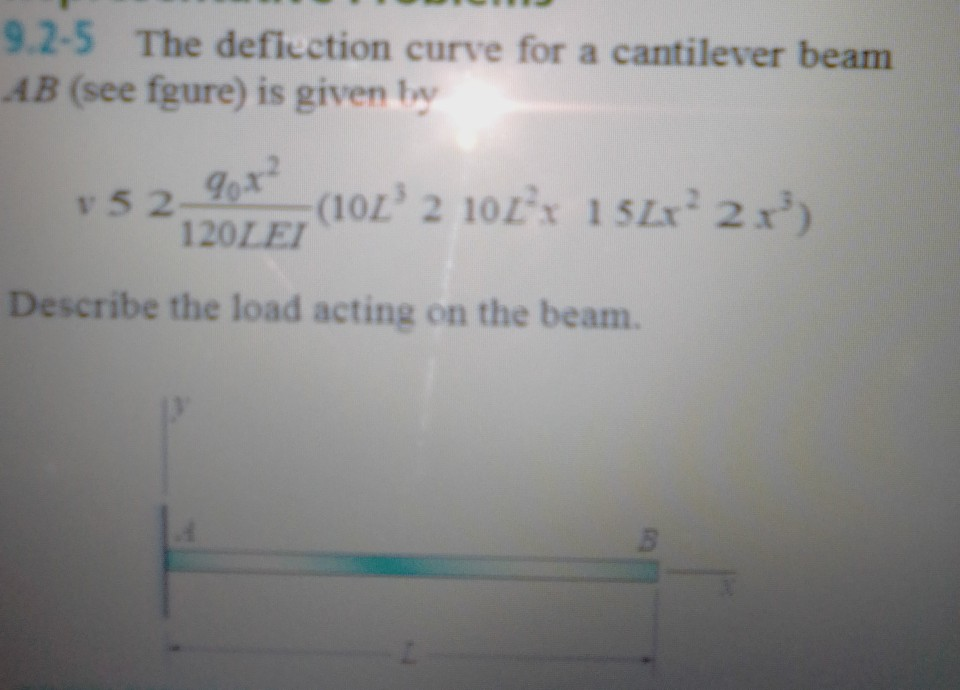Strength of Materials IV 9.2-5 The defiuction curve for a cantilever beam AB (see fgure) is given b 120LEI Describe the load acting on the beam. 2 .3-6 Calculate the maximum deflection dma of a uniformly loaded simple beam if the span length L 5 2.0 m, the intensity of the uniform load g 5 2.0 kN/m, and the maximum bending stress s 5 60 MPa. rn X The cross section of the beam is square, and the material is...

• ### 2OMNI'm 12 Om >x Given a rectangular cantilever steel beam with height h = 250 cm...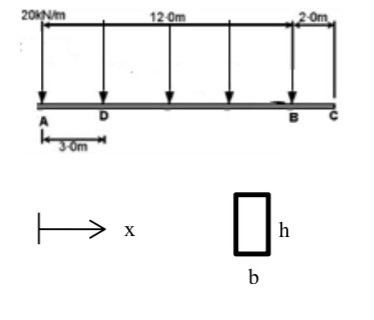2OMNI'm 12 Om >x Given a rectangular cantilever steel beam with height h = 250 cm and width b = 60 cm subjected to the triangularly distributed load shown: a. Sketch the beam shear and moment resultant loads as a function of x between 0 and L. b. Find the resultant loads (M, and V) at sections A and D. c. Determine the ratio of maximum transverse shear stress to maximum bending stress at both A and D. d. Calculate...

• ### A beam may have zero shear stress at a section but may not have zero deflection;...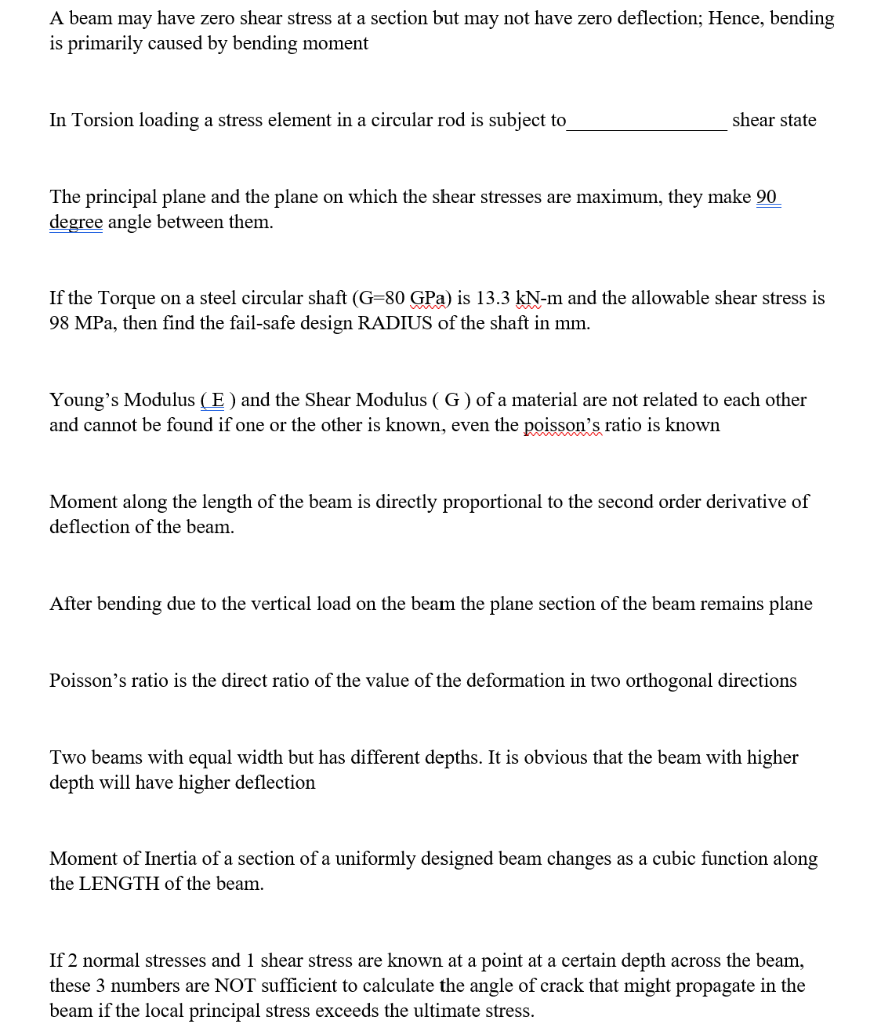A beam may have zero shear stress at a section but may not have zero deflection; Hence, bending is primarily caused by bending moment In Torsion loading a stress element in a circular rod is subject to shear state The principal plane and the plane on which the shear stresses are maximum, they make 90 degree angle between them. If the Torque on a steel circular shaft (G=80 GPa) is 13.3 kN-m and the allowable shear stress is 98 MPa,...

• ### Q2. A simply supported beam AB (Figure 2) supports a uniformly distributed load of q =...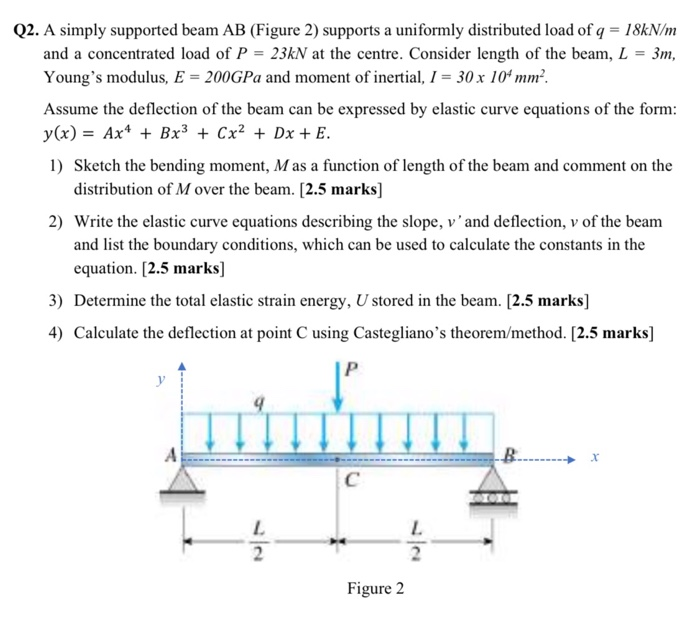Q2. A simply supported beam AB (Figure 2) supports a uniformly distributed load of q = 18kN/m and a concentrated load of P = 23kN at the centre. Consider length of the beam, L = 3m, Young's modulus, E = 200GPa and moment of inertial, I = 30 x 10 mm-. Assume the deflection of the beam can be expressed by elastic curve equations of the form: y(x) = Ax4 + Bx3 + Cx2 + Dx + E. 1) Sketch...